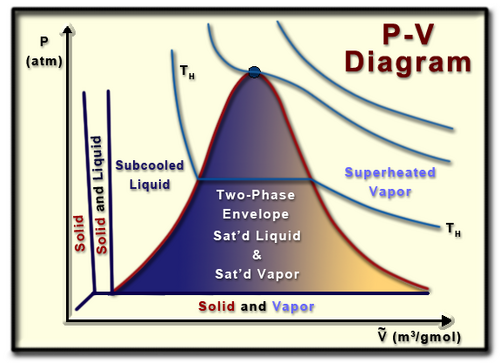Redirected from Pressure volume diagram

# Pure Substances

A pure substance is one that is homogeneous (i.e., it has the same chemical composition throughout the substance), and it can exist in three phases (gas, liquid, and solid). Properties of pure substances can help in understanding thermodynamic principles.

Common properties of pure substances include: temperature, pressure, volume, mass, density, specific volume, energy, enthalpy, and entropy. These properties can be categorized as either extensive or intensive. An intensive property is one which does not depend on the size of the system. Temperature is an example. Extensive properties are those which do depend on the size of the system. Examples include mass and volume.

Important terms when dealing with pure substances include:

vaporization, which is the point where a liquid changes into a vapor phase
condensation, which is the point that a vapor changes into a liquid phase (and is the same point as for vaporization)
melting, which is the point where a solid turns into a liquid
freezing, which is the point that a liquid turns into a solid
sublimation, which is the point that a solid turns directly into a vapor
desublimation, which is the point that a vapor turns directly into a solid (unusual but apparently it happens)
critical point, is the point where the saturated vapor and saturated liquid curves meet and become one and the same.
Triple point, is the point where the solid, liquid, and gas phases all exist at the same time at equilibrium.A P-V diagram. As the molar volume increases pressure generally decreases.

## P-v diagram specifics

A pressure volume diagram (or a P-V diagram) is a visual representation of pressure and volume of a certain pure substance (a substance that is homogeneous). Understanding a P-V diagram is helpful in understanding the properties of pure substances, an important aspect of thermodynamics.

Beginning at the top of the graph, the critical point is that location where there is no difference between the gas and liquid phase of a substance. At this point the saturated liquid and the saturated vapor become the same fluid, leading to the molar volume of the saturated liquid and of the saturated vapor to become equal.

The left side of the bell curve represents the saturated liquid line, while the right side of the bell curve represents the saturated vapor line. A saturated liquid or vapor would lie on their appropriate lines, and is exactly where a liquid would saturate or vaporize at an existing pressure. Inside of the bell curve is the so-called two phase envelope, where the liquid and gaseous phases of the substance exist at the same time. In this state a substance is said to have some sort of quality (x), where the percent quality of a substance is the ratio of amount of saturated vapor to the total mass of the substance. Mathematically quality can be defined as:

```          mg
x  = --------------
mwhere mg= mass of the gas and m= the mass of the whole substance
```

Quality can never exceed 100%. In the two-phase envelope it is important to realize that temperature and pressure are dependent. When determining a substance's state, pressure and temperature cannot be specified concurrently.

## Equations

For pure substances there are a few important equations which can be understood from the P-v graph, or its variation in a T-v (temperature-volume), P-T (pressure-temperature) graph, and so on.

To determine the total volume of the mixture, first recognize that this is the sum of the volume occupied by the liquid and the sum of the volume occupied by the gas. This can be defined as:

mv = mfvf+mgvg

specific volume can be defined as:

v = vf+x*(vg-vf)

Finally, recognize that the percent liquid by mass can be understood as 100(1-x) while the percentage vapor can be understood as 100x.

## T-v diagram

A T-v diagram demonstrates the relationship of a pure substance between temperature and volume. The curves on this diagram are isobars, or lines of constant pressure. Under the two phase envelope, although the substance has some sort of quality the molar volume of a saturated vapor or liquid at a certain pressure can be read straight off the graph. That is, the properties of saturated liquids and saturated vapors are independent of quality.

On this diagram, to the left of the two phase envelope the substance would be a subcooled liquid (because the temperature would be less than the saturation temperature). To the right of the two phase envelope the substance would be a superheated vapor (because the temperature would be greater than the saturation temperature).

## P-T diagram

A P-T diagram shows the relationship of a pure substance between pressure and temperature. This diagram displays the properties of a substance that expands upon freezing (such as water).

As can be noted from this diagram, the liquid exists at a pressure above the vapor (the vapor pressure). As such the liquid can be referred to as a compressed liquid. Following the arrows on the diagram demonstrates what needs to be done in order for a phase change to occur. For instance, let's say you had water existing in the middle of the solid range. If you kept pressure constant and solely raised the temperature, the solid would melt into a liquid. Likewise, for most of the liquid region if pressure was decreased while temperature was kept constant, the liquid would vaporize into a gas.